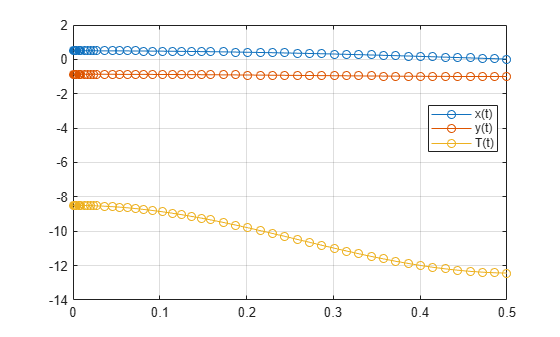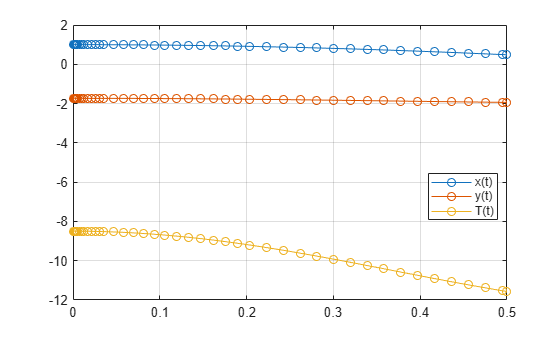# Solve Semilinear DAE System

This workflow is an alternative workflow to solving semilinear differential algebraic equations (DAEs), used only if `reduceDAEIndex` failed in the standard workflow with the warning message: `The index of the reduced DAEs is larger than 1. [daetools::reduceDAEIndex]`. For the standard workflow, see Solve Differential Algebraic Equations (DAEs).

Complete steps 1 and 2 in Solve Differential Algebraic Equations (DAEs) before beginning other steps. Then, in step 3, if `reduceDAEIndex` fails, reduce the differential index using `reduceDAEToODE`. The advantage of `reduceDAEToODE` is that it reliably reduces semilinear DAEs to ODEs (DAEs of index `0`). However, this function is slower and works only on semilinear DAE systems. `reduceDAEToODE` can fail if the system is not semilinear.

To solve your DAE system, complete these steps.

### Step 1. Specify Equations and Variables

The DAE system of equations is:

`$\begin{array}{l}m\frac{{d}^{2}x}{d{t}^{2}}=T\left(t\right)\frac{x\left(t\right)}{r}\\ m\frac{{d}^{2}y}{d{t}^{2}}=T\left(t\right)\frac{y\left(t\right)}{r}-mg\\ x{\left(t\right)}^{2}+y{\left(t\right)}^{2}={r}^{2}\end{array}$`

Specify independent variables and state variables by using `syms`.

`syms x(t) y(t) T(t) m r g`

Specify equations by using the == operator.

```eqn1 = m*diff(x(t), 2) == T(t)/r*x(t); eqn2 = m*diff(y(t), 2) == T(t)/r*y(t) - m*g; eqn3 = x(t)^2 + y(t)^2 == r^2; eqns = [eqn1 eqn2 eqn3];```

Place the state variables in a column vector. Store the number of original variables for reference.

```vars = [x(t); y(t); T(t)]; origVars = length(vars);```

### Step 2. Reduce Differential Order

The differential order of a DAE system is the highest differential order of its equations. To solve DAEs using MATLAB, the differential order must be reduced to `1`. Here, the first and second equations have second-order derivatives of `x(t)` and `y(t)`. Thus, the differential order is `2`.

Reduce the system to a first-order system by using `reduceDifferentialOrder`. The `reduceDifferentialOrder` function substitutes derivatives with new variables, such as `Dxt(t)` and `Dyt(t)`. The right side of the expressions in `eqns` is `0`.

`[eqns,vars] = reduceDifferentialOrder(eqns,vars)`
```eqns =  ```
```vars =  $\left(\begin{array}{c}x\left(t\right)\\ y\left(t\right)\\ T\left(t\right)\\ \mathrm{Dxt}\left(t\right)\\ \mathrm{Dyt}\left(t\right)\end{array}\right)$```

### Step 3. Reduce Differential Index with `reduceDAEToODE`

To reduce the differential index of the DAEs described by `eqns` and `vars`, use `reduceDAEToODE`. To reduce the index, `reduceDAEToODE` adds new variables and equations to the system. `reduceDAEToODE` also returns constraints, which are conditions that help find initial values to ensure that the resulting ODEs are equal to the initial DAEs.

`[ODEs,constraints] = reduceDAEToODE(eqns,vars)`
```ODEs =  ```
```constraints =  $\left(\begin{array}{c}-2 m r {\mathrm{Dxt}\left(t\right)}^{2}-2 m r {\mathrm{Dyt}\left(t\right)}^{2}-2 T\left(t\right) {x\left(t\right)}^{2}-2 T\left(t\right) {y\left(t\right)}^{2}+2 g m r y\left(t\right)\\ {r}^{2}-{x\left(t\right)}^{2}-{y\left(t\right)}^{2}\\ 2 \mathrm{Dxt}\left(t\right) x\left(t\right)+2 \mathrm{Dyt}\left(t\right) y\left(t\right)\end{array}\right)$```

### Step 4. ODEs to Function Handles for ode15s and ode23t

From the output of `reduceDAEToODE`, you have a vector of equations in `ODEs` and a vector of variables in `vars`. To use `ode15s` or `ode23t`, you need two function handles: one representing the mass matrix of the ODE system, and the other representing the vector containing the right sides of the mass matrix equations. These function handles are the equivalent mass matrix representation of the ODE system where M(t,y(t))y’(t) = f(t,y(t)).

Find these function handles by using `massMatrixForm` to get the mass matrix `massM` (M in the equation) and right sides `f`.

`[massM,f] = massMatrixForm(ODEs,vars)`
```massM =  $\left(\begin{array}{ccccc}-1& 0& 0& 0& 0\\ 0& -1& 0& 0& 0\\ 0& 0& 0& m& 0\\ 0& 0& 0& 0& m\\ -4 T\left(t\right) x\left(t\right)& 2 g m r-4 T\left(t\right) y\left(t\right)& -2 {x\left(t\right)}^{2}-2 {y\left(t\right)}^{2}& -4 m r \mathrm{Dxt}\left(t\right)& -4 m r \mathrm{Dyt}\left(t\right)\end{array}\right)$```
```f =  $\left(\begin{array}{c}-\mathrm{Dxt}\left(t\right)\\ -\mathrm{Dyt}\left(t\right)\\ \frac{T\left(t\right) x\left(t\right)}{r}\\ \frac{T\left(t\right) y\left(t\right)-g m r}{r}\\ 0\end{array}\right)$```

The equations in `ODEs` can contain symbolic parameters that are not specified in the vector of variables `vars`. Find these parameters by using `setdiff` on the output of `symvar` from `ODEs` and `vars`.

```pODEs = symvar(ODEs); pvars = symvar(vars); extraParams = setdiff(pODEs, pvars)```
`extraParams = $\left(\begin{array}{ccc}g& m& r\end{array}\right)$`

The extra parameters that you need to specify are the mass `m`, radius `r`, and gravitational constant `g`.

Convert `massM` and `f` to function handles using `odeFunction`. Specify the extra symbolic parameters as additional inputs to `odeFunction`.

```massM = odeFunction(massM, vars, m, r, g); f = odeFunction(f, vars, m, r, g);```

The rest of the workflow is purely numerical. Set the parameter values and substitute the parameter values in `DAEs` and `constraints`.

```m = 1; r = 1; g = 9.81; ODEsNumeric = subs(ODEs); constraintsNumeric = subs(constraints);```

Create the function handle suitable for input to `ode15s` or `ode23s`.

```M = @(t,Y) massM(t,Y,m,r,g); F = @(t,Y) f(t,Y,m,r,g);```

### Step 5. Initial Conditions for `ode15s` and `ode23t`

The solvers require initial values for all variables in the function handle. Find initial values that satisfy the equations by using the MATLAB® `decic` function. The `decic` accepts guesses (which might not satisfy the equations) for the initial conditions and tries to find satisfactory initial conditions using those guesses. `decic` can fail, in which case you must manually supply consistent initial values for your problem.

First, check the variables in `vars`.

`vars`
```vars =  $\left(\begin{array}{c}x\left(t\right)\\ y\left(t\right)\\ T\left(t\right)\\ \mathrm{Dxt}\left(t\right)\\ \mathrm{Dyt}\left(t\right)\end{array}\right)$```

Here, `Dxt(t)` is the first derivative of `x(t)`, and so on. There are 5 variables in a `5`-by-`1` vector. Therefore, guesses for initial values of variables and their derivatives must also be `5`-by-`1` vectors.

Assume the initial angular displacement of the pendulum is 30° or `pi/6`, and the origin of the coordinates is at the suspension point of the pendulum. Given that we used a radius `r` of `1`, the initial horizontal position `x(t)` is `r*sin(pi/6)`. The initial vertical position `y(t)` is `-r*cos(pi/6)`. Specify these initial values of the variables in the vector `y0est`.

Arbitrarily set the initial values of the remaining variables and their derivatives to `0`. These are not good guesses. However, they suffice for this problem. In your problem, if `decic` errors, then provide better guesses and refer to the `decic` page.

```y0est = [r*sin(pi/6); -r*cos(pi/6); 0; 0; 0]; yp0est = zeros(5,1);```

Create an option set that contains the mass matrix `M` of the system and specifies numerical tolerances for the numerical search.

`opt = odeset('Mass', M, 'RelTol', 10.0^(-7), 'AbsTol' , 10.0^(-7));`

Find initial values consistent with the system of ODEs and with the algebraic constraints by using `decic`. The parameter `[1,0,0,0,1]` in this function call fixes the first and the last element in `y0est`, so that `decic` does not change them during the numerical search. Here, this fixing is necessary to ensure `decic` finds satisfactory initial conditions.

```[y0, yp0] = decic(ODEsNumeric, vars, constraintsNumeric, 0,... y0est, [1,0,0,0,1], yp0est, opt)```
```y0 = 5×1 0.5000 -0.8660 -8.4957 0 0 ```
```yp0 = 5×1 0 0 0 -4.2479 -2.4525 ```

Now create an option set that contains the mass matrix `M` of the system and the vector `yp0` of consistent initial values for the derivatives. You will use this option set when solving the system.

`opt = odeset(opt, 'InitialSlope', yp0);`

### Step 6. Solve an ODE System with `ode15s` or `ode23t`

Solve the system integrating over the time span `0``t``0.5`. Add the grid lines and the legend to the plot. Use `ode23s` by replacing `ode15s` with `ode23s`.

```[tSol,ySol] = ode15s(F, [0, 0.5], y0, opt); plot(tSol,ySol(:,1:origVars),'-o') for k = 1:origVars S{k} = char(vars(k)); end legend(S, 'Location', 'Best') grid on```Solve the system for different parameter values by setting the new value and regenerating the function handle and initial conditions.

Set `r` to `2` and repeat the steps.

```r = 2; ODEsNumeric = subs(ODEs); constraintsNumeric = subs(constraints); M = @(t,Y) massM(t,Y,m,r,g); F = @(t,Y) f(t,Y,m,r,g); y0est = [r*sin(pi/6); -r*cos(pi/6); 0; 0; 0]; opt = odeset('Mass', M, 'RelTol', 10.0^(-7), 'AbsTol' , 10.0^(-7)); [y0, yp0] = decic(ODEsNumeric, vars, constraintsNumeric, 0,... y0est, [1,0,0,0,1], yp0est, opt); opt = odeset(opt, 'InitialSlope', yp0);```

Solve the system for the new parameter value.

```[tSol,ySol] = ode15s(F, [0, 0.5], y0, opt); plot(tSol,ySol(:,1:origVars),'-o') for k = 1:origVars S{k} = char(vars(k)); end legend(S, 'Location', 'Best') grid on```